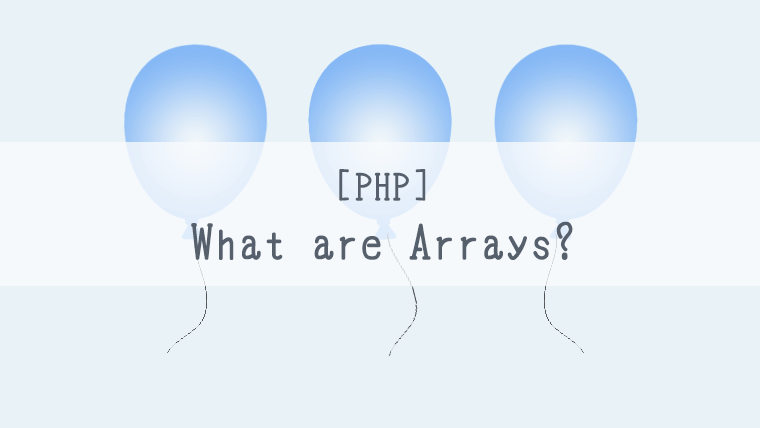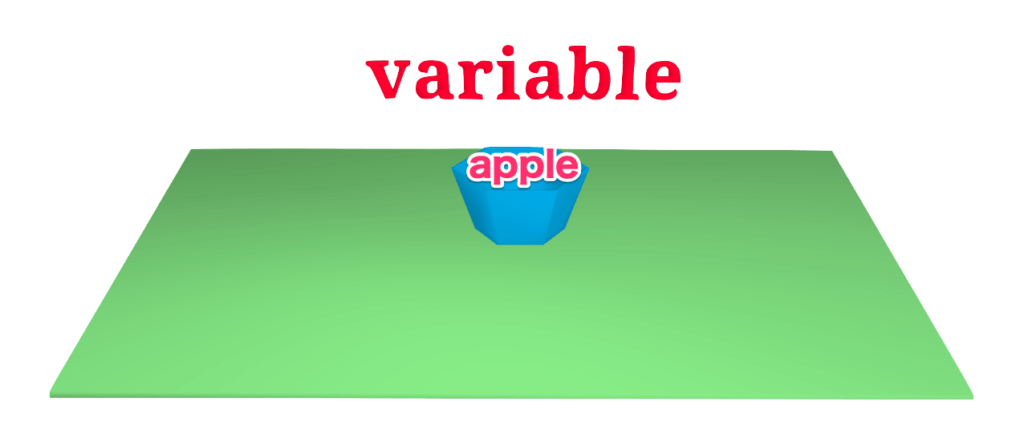Programming Lessons

# [PHP]What are Arrays?[Programming for Kids and Above #4]Hello!

I’m kat, the manager of ‘kat’s blog.’

This time, which is the fourth installment of the ‘Programming Lessons’ series, I will be talking about arrays.

In my last article, we tried entering one value in one variable, but actually you can also enter multiple values.

Let’s learn how to do that this time!

## What we will use this time

The programming language we will use this time

This time we will use the following programming language to write out a program.

・PHP

The programming environment we will use this time

Let’s use the paiza website which is listed below for executing the program.

For those who are doing this for the first time, the way to use it is listed in the page below, so please check it out.How to use paiza.io[Basics][Programming with Your Browser][PHP]Hello, I'm kat, the manager of 'kat's blog.' This time I would like ...

## What are arrays?

I mentioned at the beginning, but arrays are variables which can hold multiple values.

### How to enter values

In the case of regular variables;

```\$number = 1;
```

you enter only one value like shown above,

however for an array,

```\$numbers = [1, 2, 3];
```

like shown above, you write in multiple values inside the [], while separating them with a ‘,’.

Also, you can write it out as,

```\$numbers = array(1, 2, 3);
```

Even though how it is written is different, the meaning is the same as when we wrote it inside the [].

### How to acquire values

When acquiring values from normal variables, usually you can do this just by writing out the variable. However, in the case of arrays you need to attach a number after the variable. This number, such as  or , needs to be the number which has the value you wish to take (the number tells which order the value is in).

In the case of regular variables;

```<?php

\$number = 1;
echo \$number;  // is the regular way to acquire variables.
```

The execution result will be displayed as ‘1’.

How to acquire values from arrays

```<?php

\$numbers = [1, 2, 3];   // in the zero position is the value '1,' in the one position is '2,' and in the two position is '3.'
echo \$numbers;       // this is acquiring the number one value '2.'
```

As some of you might have noticed already, the numbers start from zero.

So, because  is selected above, the number one value, ‘2’ will be displayed on the screen.

The [0(zero)], , which is put after an array, is called a ‘key‘ in programming terminology.

For instance, the keys are 0,1,2 in the case of \$numbers = [1, 2, 3].

This phrase is used very often, so let’s try to remember it!

### Let’s enter letters into a value

You can put in letters as well as numbers into variables.

The way to do this is almost the same as if you were putting in numbers, but you will need to surround the value with ‘(quotations) or with “(double quotations).

Now then, let’s try entering letters both into a variable as well as in an array.

In the case of regular variables,

```<?php

\$fruit = "apple";
echo \$fruit;         // "apple" is how it will be displayed.
```In the case of an array;

```<?php

\$fruits = ["apple", "orange", "banana", "strawberry"];
echo \$fruits;        // "orange" is how it would be displayed.
```## Final thoughts

We talked about arrays this time. They are used just as much as regular variables, so please make sure to remember them.

Next time, I’ll be talking about associative arrays. They are easier to use than arrays, so please look forward to it!

See you next time!